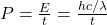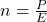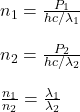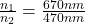## A red laser with a wavelength of 670 nmnm and a blue laser with a wavelength of 470 nmnm emit laser beams with the same light power. How do

Question

A red laser with a wavelength of 670 nmnm and a blue laser with a wavelength of 470 nmnm emit laser beams with the same light power. How do their rates of photon emission compare

in progress 0
5 months 2021-08-25T19:14:49+00:00 1 Answers 2 views 0

## Answers ( )

red laser emits 1.42 times the number of photons of the blue laser, per unit of time

Explanation:

In order to calculate the rates of photon emission for both wavelengths, you take into account that the power of the light is given by the following formula:(1)

That is, the power is the energy per time.

h: Planck’s constant

c: speed of light

λ: wavelength of the light

The number of photons emitted per unit of time is given by:P: power of the light

E: energy of the light

For the two wavelengths you have(2)

Where you have use that P1=P2

Finally, you replace the values of the wavelengths in the equation (2):$$\ = 1.42\frac{photons}{s}$$

Then, the red laser emits 1.42 times the number of photons emited by the blue laser